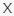# Math Trivia Quiz #2 TriviaBandit

Q 1. What is the correct order of operations for solving equations?Choices:

PARENTHESES, EXPONENTS, MULTIPLICATION, DIVISION, ADDITION, SUBTRACTION
PARENTHESES, EXPONENTS, ADDITION, SUBSTRACTION, MULTIPLICATION, DIVISION
THE ORDER IN WHICH THE OPERATIONS ARE WRITTEN.

PARENTHESES, EXPONENTS, MULTIPLICATION, DIVISION, ADDITION, SUBTRACTION

Q 2. Which of these numbers is greater?Choices:

50 X 0.2 X 5
100 X 0.09
1 X 10
49

50 X 0.2 X 5

Q 3. How many sides does an Hexagon have?Choices:

5
7
8
6

6

Q 4. Ted pays \$2.25 for a one fourth of a yard of fabric. How much does a yard of fabric cost?Choices:

9
6.75
5.25
4.5

9

Q 5. Solve this: (34 + 26) x 4 + 18Choices:

1320
678
312
222

222

Q 6. What is the sum of these numbers: -10, -4, -6, 2Choices:

-18
-22
14
22

-18

Q 7. The sum of 5x + 2y and 2x – y is:Choices:

7X + Y
7X + 2Y
3X + 2Y
3X + Y

7X + Y

Q 8. What is the name of an angle equal to 90°?Choices:

PERFECT ANGLE
RIGHT ANGLE
STRAIGHT ANGLE
OPEN ANGLE

RIGHT ANGLE

Q 9. Anna is 54 years old and her mother is 80, how many years ago was Anna’s mother three times her age?Choices:

41 YEARS AGO
49 YEARS AGO
28 YEARS AGO
30 YEARS AGO

41 YEARS AGO

Q 10. What is the square root of 144?Choices:

14
22
12
13